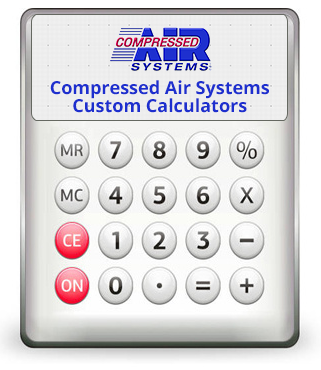# Custom CalculatorsCompressed Air Systems has a number of web calculators we put together for your use, which are available for download by filling out the short form to the right. In this collection, you'll receive the following:

• Additional Capacity Calculator
• Atmospheric Pressure Calculator
• Event Storage Calculator
• Pressure Drop & Flow Velocity in Piping Calculator
• Pump Up Time Calculator
• SCFM to ACFM Converter Calculator
• Vacuum System Sizing Calculator
• Storage in Piping Calculator